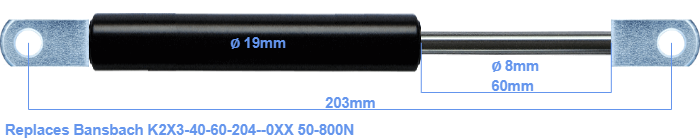# Replacement for Bansbach K2X3-40-60-204–0XX 50-800N

\$28.16

Replacement gas spring for the Bansbach K2X3-40-60-204--0XX 50-800 Newton. The eye has a thickness of 10mm and a hole diameter of 8.1mm. Brand: HAHN, a Stabilus company.

Please note:
• Mounting part tube: The thickness of the eye of this replacement is 10mm instead of 14mm.
• Mounting part rod : The thickness of the eye of this replacement is 10mm instead of 12mm.
•  Force Choose an option50 Newton60 Newton80 Newton100 Newton120 Newton140 Newton150 Newton160 Newton180 Newton200 Newton220 Newton240 Newton250 Newton260 Newton280 Newton300 Newton320 Newton340 Newton350 Newton360 Newton380 Newton400 Newton420 Newton440 Newton450 Newton460 Newton480 Newton500 Newton520 Newton540 Newton550 Newton560 Newton580 Newton600 Newton620 Newton640 Newton650 Newton660 Newton680 Newton700 Newton720 Newton740 Newton750 Newton760 Newton780 Newton800 NewtonClearThe diameter of the cylinder of this gas spring is 19 mm. The diameter of the rod is 8 millimeter. The rod (the chrome part) is 60 mm long. The total length of this replacement is 203 millimeter. This is the distance between the centers of the mounting parts. The distance from thread to thread (without mounting parts) is 165 mm. This gas spring (K2X3-40-60-204--0XX) has a force of 50-800 Newton. This is not an original gas spring from Bansbach, but a so called replacement gas spring. HAHN Gasfedern, a Stabilus subsidiary, is responsible for the production of this replacement gas spring. The gas spring has the brand HAHN. The force (50-800 N) and dimensions are almost identical to the original.